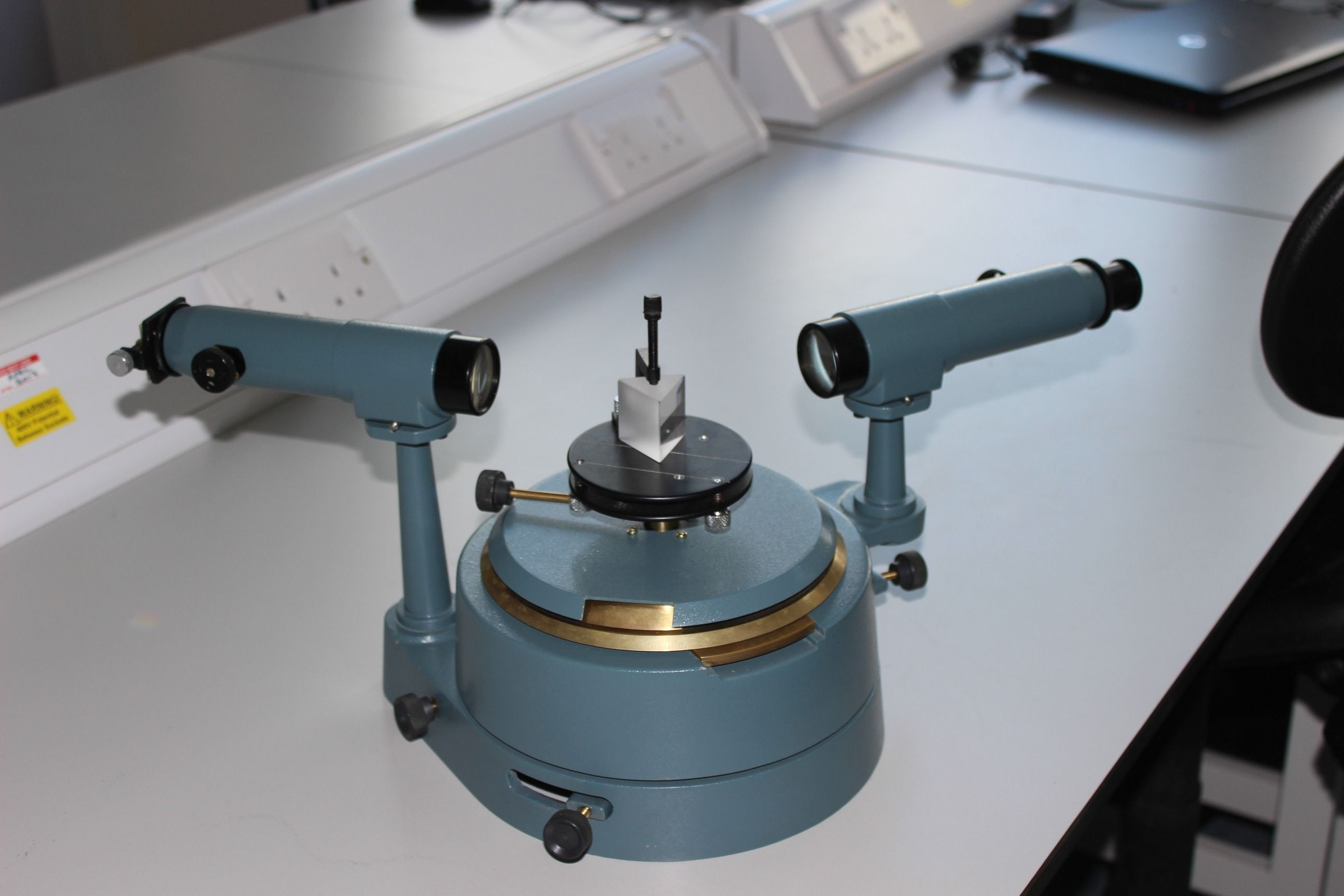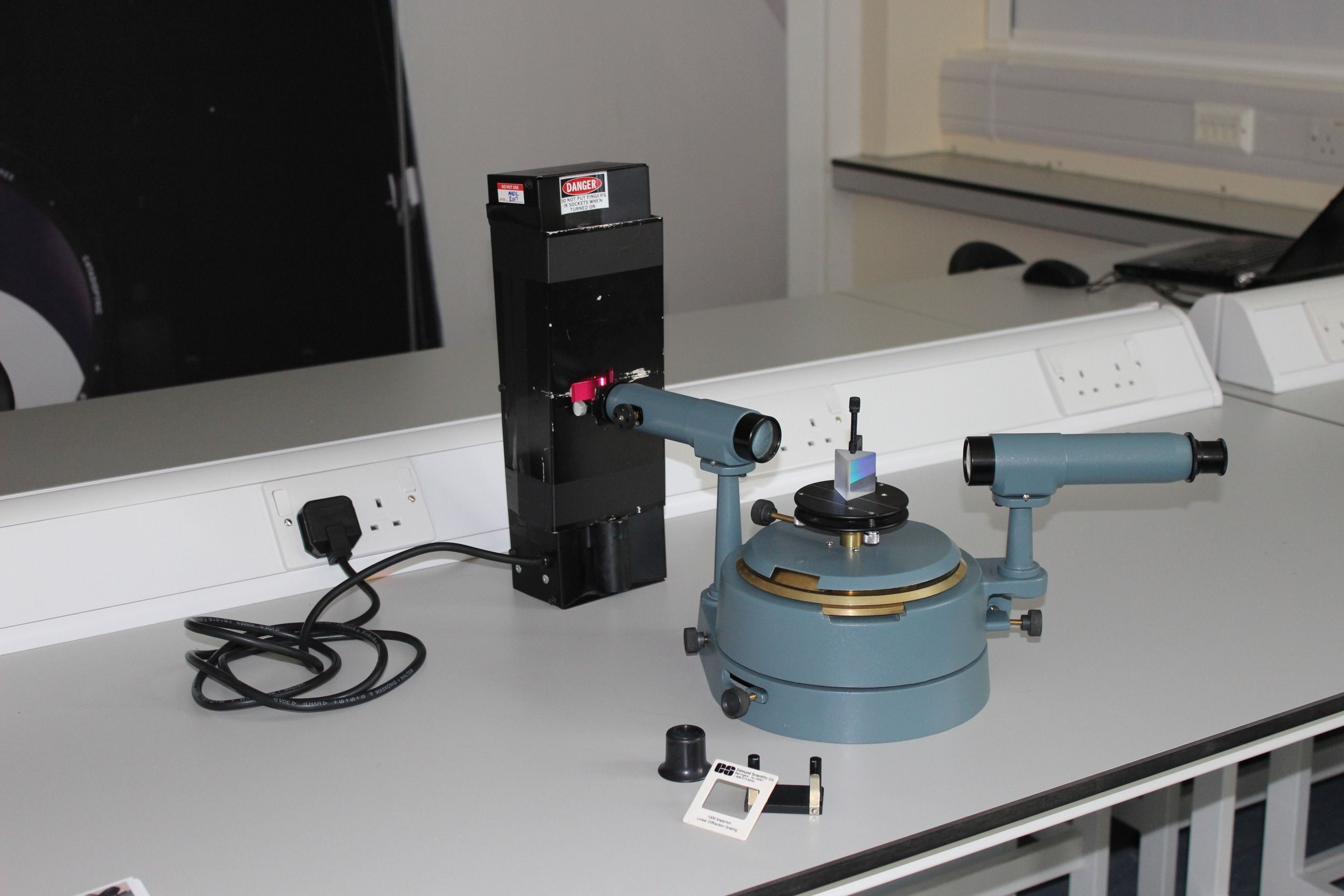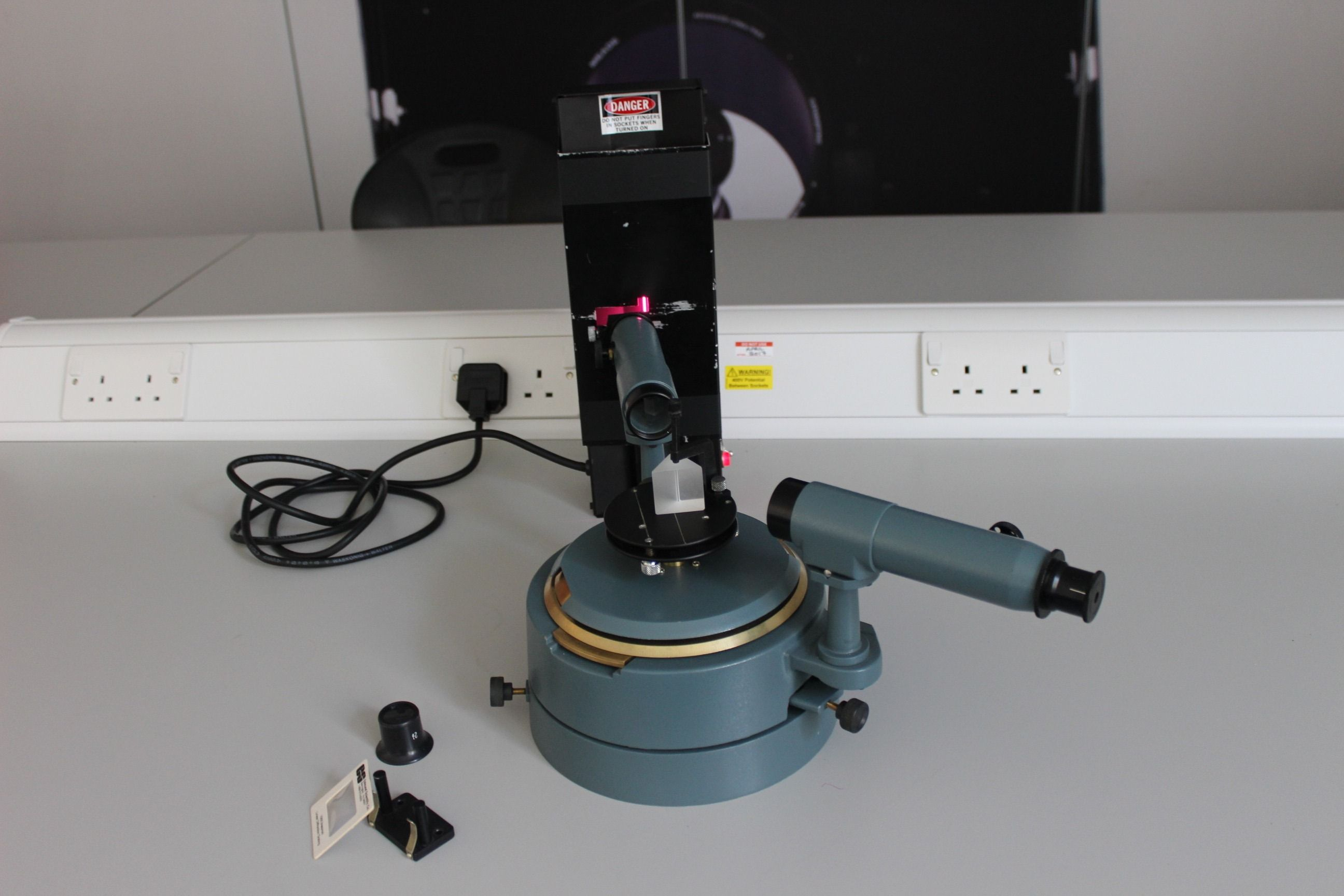The prism spectrometerEquipment provided for the sessionEquipment provided for the session

## Prism Spectrometer

### In this session you will have:

Gained a proficiency in the reading of vernier scales

Learnt a method for the measurement of refractive index

Understood the accuracy and precision limits to which refractive index may be measured

Kept, and submitted, a clearly laid out set of laboratory notes

### Introduction

Accurate measurement of the refractive index of materials can be obtained using a prism made out of the material of interest. Rays passing though the prism deviate because of refraction. Simple ray (geometrical) optics and the use of Snell's law show that the index is directly related to the minimum angular deviation of a ray that passes through the prism at different angles of incidence. You can measure this minimum deviation directly for any ray that comprises a single wavelength of light (a monochromatic beam).

A cadmium lamp provides a few discrete wavelengths and for each wavelength the minimum deviation angle will be different. This is because the prism refractive index varies with wavelength (the property known as dispersion) and so you will also be able to measure this property as well.

There is an ISE where you can practice this experiment before your session. It can be found here.

### Script

The script for this experiment can be found in the lab script book or on DUO.